# Mesh Parameterization¶

Important: Please read the installation page for details about how to install the toolboxes. $\newcommand{\dotp}{\langle #1, #2 \rangle}$ $\newcommand{\enscond}{\lbrace #1, #2 \rbrace}$ $\newcommand{\pd}{ \frac{ \partial #1}{\partial #2} }$ $\newcommand{\umin}{\underset{#1}{\min}\;}$ $\newcommand{\umax}{\underset{#1}{\max}\;}$ $\newcommand{\umin}{\underset{#1}{\min}\;}$ $\newcommand{\uargmin}{\underset{#1}{argmin}\;}$ $\newcommand{\norm}{\|#1\|}$ $\newcommand{\abs}{\left|#1\right|}$ $\newcommand{\choice}{ \left\{ \begin{array}{l} #1 \end{array} \right. }$ $\newcommand{\pa}{\left(#1\right)}$ $\newcommand{\diag}{{diag}\left( #1 \right)}$ $\newcommand{\qandq}{\quad\text{and}\quad}$ $\newcommand{\qwhereq}{\quad\text{where}\quad}$ $\newcommand{\qifq}{ \quad \text{if} \quad }$ $\newcommand{\qarrq}{ \quad \Longrightarrow \quad }$ $\newcommand{\ZZ}{\mathbb{Z}}$ $\newcommand{\CC}{\mathbb{C}}$ $\newcommand{\RR}{\mathbb{R}}$ $\newcommand{\EE}{\mathbb{E}}$ $\newcommand{\Zz}{\mathcal{Z}}$ $\newcommand{\Ww}{\mathcal{W}}$ $\newcommand{\Vv}{\mathcal{V}}$ $\newcommand{\Nn}{\mathcal{N}}$ $\newcommand{\NN}{\mathcal{N}}$ $\newcommand{\Hh}{\mathcal{H}}$ $\newcommand{\Bb}{\mathcal{B}}$ $\newcommand{\Ee}{\mathcal{E}}$ $\newcommand{\Cc}{\mathcal{C}}$ $\newcommand{\Gg}{\mathcal{G}}$ $\newcommand{\Ss}{\mathcal{S}}$ $\newcommand{\Pp}{\mathcal{P}}$ $\newcommand{\Ff}{\mathcal{F}}$ $\newcommand{\Xx}{\mathcal{X}}$ $\newcommand{\Mm}{\mathcal{M}}$ $\newcommand{\Ii}{\mathcal{I}}$ $\newcommand{\Dd}{\mathcal{D}}$ $\newcommand{\Ll}{\mathcal{L}}$ $\newcommand{\Tt}{\mathcal{T}}$ $\newcommand{\si}{\sigma}$ $\newcommand{\al}{\alpha}$ $\newcommand{\la}{\lambda}$ $\newcommand{\ga}{\gamma}$ $\newcommand{\Ga}{\Gamma}$ $\newcommand{\La}{\Lambda}$ $\newcommand{\si}{\sigma}$ $\newcommand{\Si}{\Sigma}$ $\newcommand{\be}{\beta}$ $\newcommand{\de}{\delta}$ $\newcommand{\De}{\Delta}$ $\newcommand{\phi}{\varphi}$ $\newcommand{\th}{\theta}$ $\newcommand{\om}{\omega}$ $\newcommand{\Om}{\Omega}$

This tour explores 2-D parameterization of 3D surfaces using linear methods.

A review paper for mesh parameterization can be found in:

M.S. Floater and K. Hormann, Surface Parameterization: a Tutorial and Survey in Advances in multiresolution for geometric modelling, p. 157-186, 2005.

K. Hormann, K. Polthier and A. Sheffer Mesh parameterization: theory and practice, Siggraph Asia Course Notes

In :
options(repr.plot.width=3.5, repr.plot.height=3.5)
options(warn=-1) # turns off warnings, to turn on: "options(warn=0)"

library(Matrix)
library(rgl)
library(geometry)
library(plotly)
library(akima)
library(plot3D)
library(network)

# Importing the libraries
for (f in list.files(path="nt_toolbox/toolbox_general/", pattern="*.R")) {
source(paste("nt_toolbox/toolbox_general/", f, sep=""))
}
for (f in list.files(path="nt_toolbox/toolbox_signal/", pattern="*.R")) {
source(paste("nt_toolbox/toolbox_signal/", f, sep=""))
}


## Conformal Laplacian¶

The conformal Laplacian uses the cotan weights to obtain an accurate discretization of the Laplace Beltrami Laplacian.

They where first introduces as a linear finite element approximation of the Laplace-Beltrami operator in:

U. Pinkall and K. Polthier, Computing discrete minimal surfaces and their conjugates Experimental Mathematics, 2(1):15-36, 1993.

First load a mesh. The faces are stored in a matrix $F = (f_j)_{j=1}^m \in \RR^{3 \times m}$ of $m$ faces $f_j \in \{1,\ldots,n\}^3$. The position of the vertices are stored in a matrix $X = (x_i)_{i=1}^n \in \RR^{3 \times n}$ of $n$ triplets of points $x_k \in \RR^3$

In :
X = read_mesh("nt_toolbox/data/nefertiti.off")$X0 F = read_mesh("nt_toolbox/data/nefertiti.off")$F0
n = dim(X)


In order to perform mesh parameterization, it is important that this mesh has the topology of a disk, i.e. it should have a single B.

First we compute the boundary $B = (i_1,\ldots,i_p)$ of the mesh. By definition, for the edges $(i_k,i_{k+1})$, there is a single adjacent face $(i_k,i_{k+1},\ell)$.

In :
B = compute_boundary(F)


Length of the boundary.

In :
p = length(B)


Display the boundary.

In :
options(repr.plot.width=3.5, repr.plot.height=5)
# plot mesh
plot_mesh(X,F)

#plot boundary
lines(X[1,B+1], X[2,B+1], col="red", lwd=3)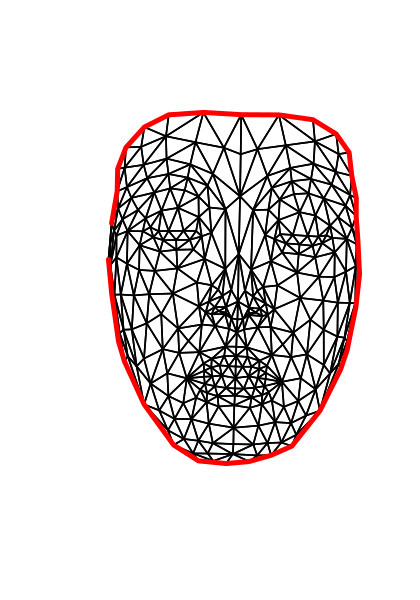The conformal Laplacian weight matrix $W \in \RR^{n \times n}$ is defined as $$W_{i,j} = \choice{ \text{cotan}(\al_{i,j}) + \text{cotan}(\be_{i,j}) \qifq i \sim j \\ \quad 0 \quad \text{otherwise}. }$$ Here, $i \times j$ means that there exists two faces $(i,j,k)$ and $(i,j,\ell)$ in the mesh (note that for B faces, one has $k=\ell$).

The angles are the angles centered as $k$ and $\ell$, i.e. $$\al_{i,j} = \widehat{x_i x_k x_j } \qandq \be_{i,j} = \widehat{x_i x_\ell x_j }.$$

Compute the conformal 'cotan' weights. Note that each angle $\alpha$ in the mesh contributes with $1/\text{tan}(\alpha)$ to the weight of the opposite edge. We compute $\alpha$ as $$\alpha = \text{acos}\pa{ \frac{\dotp{u}{v}}{\norm{u}\norm{v}} }$$ where $u \in \RR^3, v \in \RR^3$ are the edges of the adjacent vertices that defines $\al$.

In :
W = Matrix(0, nrow=n, ncol=n, sparse=TRUE)

for (i in (0:2))
{
i2 = (i+1)%%3
i3 = (i+2)%%3
u = X[,(F+1)[i2+1,]] - X[,(F+1)[i+1,]]
v = X[,(F+1)[i3+1,]] - X[,(F+1)[i+1,]]
u = u/rbind(sqrt(colSums(u**2)), sqrt(colSums(u**2)),sqrt(colSums(u**2)));
v = v/rbind(sqrt(colSums(v**2)), sqrt(colSums(v**2)),sqrt(colSums(v**2)));
alpha = 1/(tan(acos(colSums(u*v))))
alpha = pmax(alpha, 10^(-2)*matrix(1,nrow=1, ncol=length(alpha)))
W = W+sparseMatrix(x=alpha, i=(F+1)[i2+1,], j=(F+1)[i3+1,], dims=c(n,n))
W = W+sparseMatrix(x=alpha, i=(F+1)[i3+1,], j=(F+1)[i2+1,], dims=c(n,n))
}


Compute the symmetric Laplacian matrix $L = D-W$ where $D = \mathrm{Diag}_i\pa{\sum_j W_{i,j}}$

In :
d = as.vector(colSums(W))
D = Diagonal(length(d),d)
L = D - W


## Fixed Boundary Harmonic Parameterization¶

The problem of mesh parameterization corresponds to finding 2-D locations $(y_i = (y_i^1,y_i^2) \in \RR^2$ for each original vertex, where $Y = (y_i)_{i=1}^n \in \RR^{2 \times n}$ denotes the flattened positions.

The goal is for this parameterization to be valid, i.e. the 2-D mesh obtained by replacing $X$ by $Y$ but keeping the same face connectivity $F$ should not contained flipped faces (all face should have the same orientation in the plane).

We consider here a linear methods, that finds the parameterization, that impose that the coordinates are harmonic inside the domain, and have fixed position on the boundary (Dirichlet conditions) $$\forall s=1,2, \quad \forall i \notin B, \quad (L y^s)_i = 0, \qandq \forall j \in B, \quad y^s_j = z_j^s.$$

In order for this method to define a valid parameterization, it is necessary that the fixed position $z_j = (z^1_j,z^2_j) \in \RR^2$ are consecutive points along a convex polygon.

Compute the fixed positions $Z=(z_j)_j$ for the vertices on $B$. Here we use a circle.

In :
p = length(B)
t = seq(from=0,to=2*pi,by=(2*pi/p))
t = t[-(p+1)]
Z = rbind(cos(t),sin(t))


Computing the parameterization requires to solve two independent linear system $$\forall s=1,2, \quad L_1 y^s = r^s$$ where $L_1$ is a modified Laplacian, the is obtained from $L$ by $$\choice{ \forall i \notin B, \quad (L_0)_{i,j} = L_{i,j} \\ \forall i \in B, \quad (L_0)_{i,i}=1, \\ \forall i \in B, \forall j \neq i, \quad (L_0)_{i,i}=0, }$$ i.e. replacing each row indexed by $B$ by a 1 on the diagonal.

In :
L1 = Matrix(L, sparse=TRUE)

L1[B+1,] = 0

for (i in (1:length(B)))
{
L1[(B + 1)[i], (B + 1)[i]] = 1
}


Set up the right hand size $R$ with the fixed position.

In :
R = Matrix(0, nrow=2, ncol=n)
R[,B+1] = Z


Solve the two linear systems.

In :
Y = Matrix(0, nrow=2, ncol=n)

Y[1,] = solve(L1,R[1,])
Y[2,] = solve(L1,R[2,])


Display the parameterization.

In :
options(repr.plot.width=4, repr.plot.height=5, colorscale=gray)

plot_mesh(as.matrix(rbind(Y,matrix(0,nrow=1,ncol=n))),F)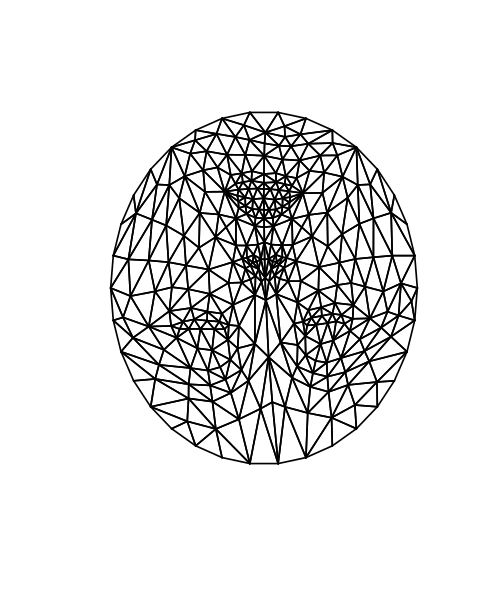## Mesh Parameterization on a Square¶

One can perform a fixed B parameterization on a square. This is useful to compute a geometry image (a color image storring the position of the vertices).

Exercise 1

Compute the fixed positions $Z$ of the points indexed by $B$ that are along a square. Warning: $p$ is not divisible by 4.

In :
source("nt_solutions/meshdeform_1_parameterization/exo1.R")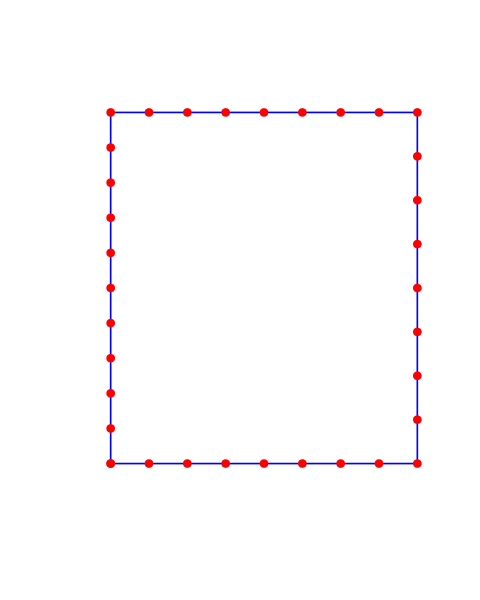In :
## Insert your code here.


Exercise 2

Compute the parameterization $Y$ on a square.

In :
source("nt_solutions/meshdeform_1_parameterization/exo2.R")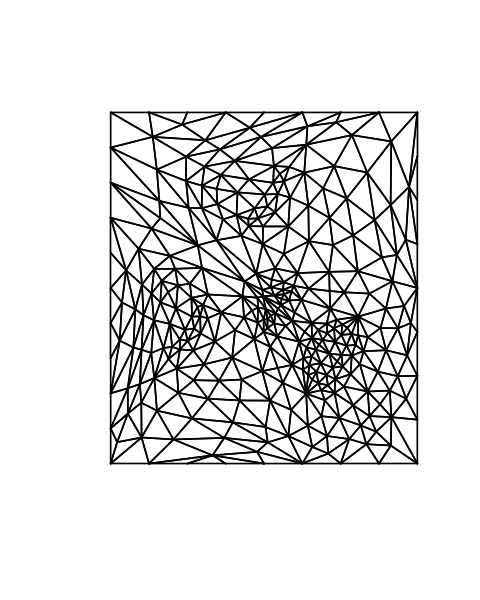In :
## Insert your code here.


Exercise 3

Shift the $B$ positions so that the eyes of the model are approximately horizontal.

In :
source("nt_solutions/meshdeform_1_parameterization/exo3.R")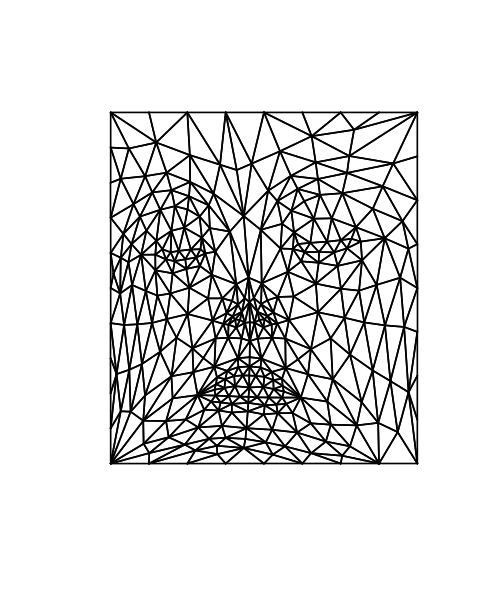In :
## Insert your code here.


## Re-align the Texture¶

To map correctly a real image on the surface, the texture needs to be aligned. We use here a simple affine mapping to map the eye and mouth of the image on the corresponding location on the surface.

Load a texture image $T$.

In :
library(Matrix)
C  =  replicate(3, runif(1000, 0, 1))
C  =  C/cbind(Matrix(rowSums(C)),Matrix(rowSums(C)),Matrix(rowSums(C)))
C  =  C[order(C[,1], C[,2], C[,3]),]
lambd = t(C)

In :
library(imager)
library(magick)

n1  =  256


Display the texture on the mesh, using the parametrization of the mesh as texture coordinates.

In :
options(repr.plot.width=6, repr.plot.height=5)

x  =  seq(from = 0, to = 1, by = 1/(dim(T)-1))

for (i in (1:100)){

px  =  Y[1,(F+1)[,i]]
py  =  Y[2, (F+1)[,i]]

points_x  =  t(lambd) %*% px
points_y  =  t(lambd) %*% py
points  =  cbind(points_x,points_y)

col  =  rescale(bicubic(x, x, T, points_x, points_y )$z) col1 = cbind(col, col, col) Px = X[1,(F+1)[,i]] Py = X[2,(F+1)[,i]] Pz = X[3,(F+1)[,i]] Points_x = t(lambd) %*% Px Points_y = t(lambd) %*% Py Points_z = t(lambd) %*% Pz points3D(matrix(Points_x), matrix(Points_y), matrix(Points_z), col=as.color(col), pch=20, box=FALSE,colkey=FALSE,cex=0.05, theta=10) par(new=TRUE) }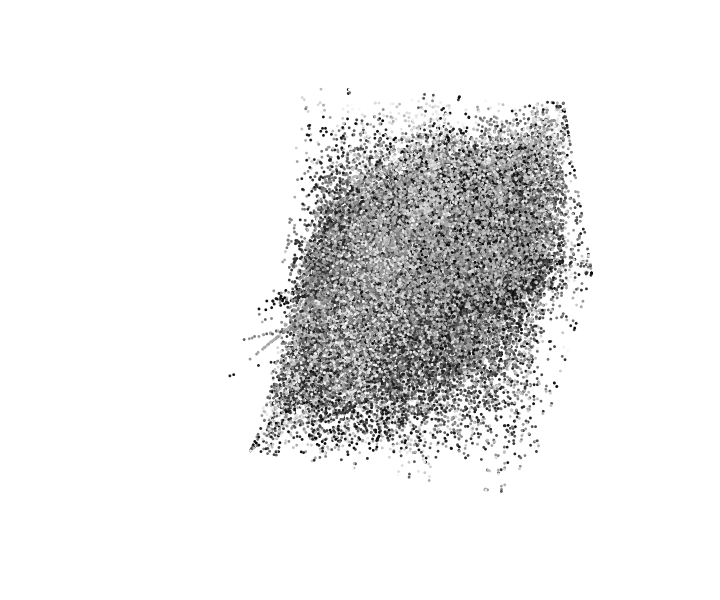Position$(u_1,u_2,u_3)$of the eyes and the mouth in the texture. In : u1 = c(267,266)*n1/512 u2 = c(267,328)*n1/512 u3 = c(355,301)*n1/512  Display. In : imageplot(T) lines(c(u1,u2,u3, u1),c(u1,u2,u3,u1), col="blue")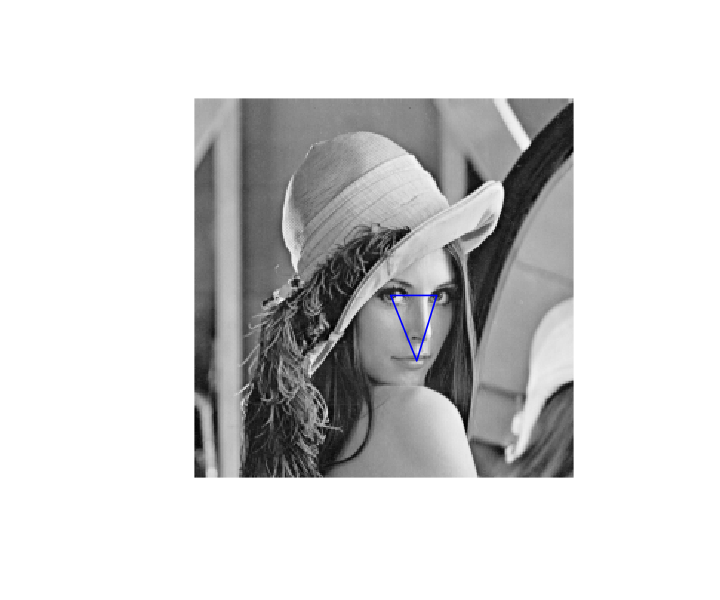Positions$(v_1,v_2,v_3)\$ of the eyes and the mouth on the parameteric domain

In :
v1  =  c(310,125)*n1/512.
v2  =  c(315,350)*n1/512.
v3  =  c(105,232)*n1/512.


Display.

In :
options(repr.plot.width=5, repr.plot.height=5)

Y_big  =  Y*(n1-1) + 1
beg  =  sort(W@i)
end  =  W@i

plot (NA, xlim=c(0,250), ylim=c(0,250), box=FALSE, clab="", ylab="", axes=FALSE)
for (i in (1:length(beg))){
lines(c(Y_big[1, (beg+1)[i]],Y_big[1,(end+1)[i]]), c(Y_big[2,(beg+1)[i]], Y_big[2,(end+1)[i]]), col="Blue", xlab="", ylab="", axes=FALSE)
}

lines (c(v1,v2,v3, v1),c(v1,v2,v3,v1), col="red")